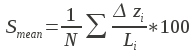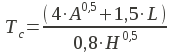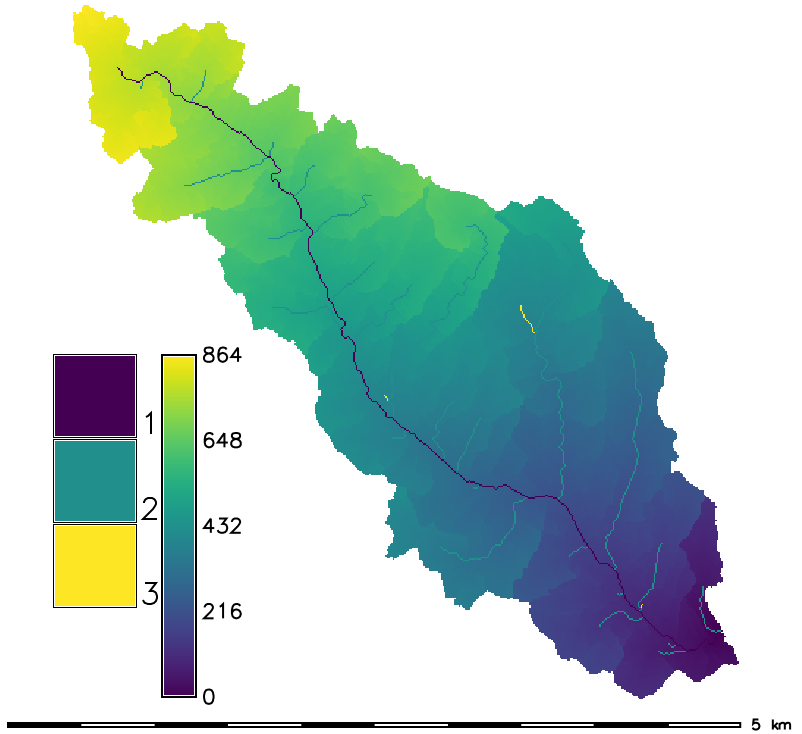## NAME

r.basin - Morphometric characterization of river basins

## KEYWORDS

raster, hydrology, watershed

## SYNOPSIS

r.basin
r.basin --help
r.basin [-ac] map=name prefix=prefix coordinates=east,north dir=name [threshold=threshold] [--overwrite] [--help] [--verbose] [--quiet] [--ui]

### Flags:

-a
Use default threshold (1km^2)
-c
No maps output
--overwrite
Allow output files to overwrite existing files
--help
Print usage summary
--verbose
Verbose module output
--quiet
Quiet module output
--ui
Force launching GUI dialog

### Parameters:

map=name [required]
Name of elevation raster map
prefix=prefix [required]
coordinates=east,north [required]
coordinates of the outlet (east,north)
dir=name [required]
Directory where the output will be found
threshold=threshold
threshold

## DESCRIPTION

r.basin generates the main morphometric parameters of the basin starting from the digital elevation model and the coordinates of the basin's closing section (outlet). Important: r.basin works in projected locations, geographic or xy-locations are not supported.

The closing section's coordinates must belong to the river network generated by r.stream.extract. It is suggested to run it beforehand. In GRASS GIS 7, r.basin has been improved to take in input coordinates not exactly belonging to the river network (but not too far from it). It basically snaps to the closest point belonging to the network. This feature is experimental and might not produce the expected result. To check if the snapped outlet is acceptable, at the end of the computation, two outlet vector maps are produced: the one with the coordinates inserted by the user and the snapped one. If the snapped outlet is not acceptable, the user should tweak the coordinates to match with the river network.

### Morphometric parameters of basin

The main parameters are:
• The coordinates of the vertices of the rectangle containing the basin.
• The center of gravity of the basin: the coordinates of the pixel nearest to the center of gravity of the geometric figure resulting from the projection of the basin on the horizontal plane.
• The area of the basin: is the area of a single cell multiplied by the number of cells belonging to the basin.
• The perimeter: is the length of the contour of the figure resulting by the projection of the basin on the horizontal plane.
• Characteristic values of elevation: the highest and the lowest altitude, the difference between them and the mean elevation calculated as the sum of the values of the cells divided by the number of the cells.
• The mean slope, calculated averaging the slope map.
• The length of the directing vector: the length of the vector linking the outlet to the center of gravity of the basin.
• The prevalent orientation: in GRASS GIS the aspect categories represent the number degrees of east and they increase counterclockwise: (90deg is North, 180 is West, 270 is South 360 is East). The aspect value 0 is used to indicate undefined aspect in flat areas with slope=0. We instead calculate the orientation as the number of degrees from north, increasing counterclockwise.
• The length of the main channel: is the length of the longest succession of segments that connect a source to the outlet of the basin.
• The mean slope of the main channel: it is calculated as followswhere N is the topological diameter, i.e. the number of links in which the main channel can be divided on the basis of the junctions.
• The circularity ratio: is the ratio between the area of the basin and the area of the circle having the same perimeter of the basin.
• The elongation ratio: is the ratio between the diameter of the circle having the same area of the basin and the length of the main channel.
• The compactness coefficient: is the ratio between the perimeter of the basin and the diameter of the circle having the same area of the basin.
• The shape factor: is the ratio between the area of the basin and the square of the length of the main channel.
• The concentration time (Giandotti, 1934):where A is the area, L the length of the main channel and H the difference between the highest and the lowest elevation of the basin.
• The mean hillslope length: is the mean of the distances calculated along the flow direction of each point not belonging to the river network from the point in which flows into the network.
• The magnitude: is the number of the branches of order 1 following the Strahler hierarchy.
• The max order: is the order of the basin, following the Strahler hierarchy.
• The number of streams: is the number of the branches of the river network.
• The total stream length: is the sum of the length of every branch.
• The first order stream frequency: is the ratio between the magnitude and the area of the basin.
• The drainage density: is the ratio between the total length of the river network and the area.
• The Horton ratios (Horton, 1945; Strahler, 1957).

### Plots

• The distance-area function, also known as Width Function: in x axis is the length and in y axis is the area.
• The hypsographic curve provides the distribution of the areas at different altitudes. Each point on the hypsographic curve has on the y-axis the altitude and on the x-axis the percentage of basin surface placed above that altitude.
• The hypsometric curve has the same shape but is dimensionless.

## EXAMPLE

North Carolina sample dataset example:
```g.region raster=elevation -p
r.basin map=elevation prefix=my_basin coord=637304.924954,218617.100523 \
dir=/tmp/my_basin threshold=1000

# visualize some results
d.mon wx0
d.rast my_basin_elevation_hack

d.rast my_basin_elevation_dist2out

d.his i=aspect h=my_basin_elevation_dist2out
```### Dependencies

• Matplotlib
• r.hypso
• r.stream.basins
• r.stream.distance
• r.stream.extract
• r.stream.order
• r.stream.snap
• r.stream.stats
• r.width.funct

## Known issues

• r.basin hasn't been designed for working in lat/long coordinates. This means that if you are working in lat/long coordinates, you need to reproject your map first in order to apply the tool.
• r.basin is designed to work in meters unit. The values that you get using feet are nonsense.
• r.basin does not handle overwrite. You need to delete the output products already created (maps, text files, figures) by hand before running it again.

Related Wiki page

## CITE AS

Di Leo Margherita, Di Stefano Massimo (2013) An Open-Source Approach for Catchment's Physiographic Characterization, Abstract H52E-06 presented at 2013 Fall Meeting, AGU, San Francisco, CA, USA 9-13 Dec. abstract

## REFERENCES

• Rodriguez-Iturbe I., Rinaldo A. (2001) Fractal River Basins, Chance and Self-Organization. Cambridge Press
• In Italian: Di Leo M., Di Stefano M., Claps P., Sole A. (2010) Caratterizzazione morfometrica del bacino idrografico in GRASS GIS (Morphometric characterization of the catchment in GRASS GIS environment), Geomatics Workbooks, n. 9

## AUTHORS

Margherita Di Leo (grass-dev AT lists DOT osgeo DOT org ), Massimo Di Stefano

## SOURCE CODE

Available at: r.basin source code (history)

Latest change: Wednesday Mar 30 10:17:35 2022 in commit: 25b0a9981b66c443a1c1af1d5f26182c93268b45

© 2003-2022 GRASS Development Team, GRASS GIS 8.2.0 Reference Manual